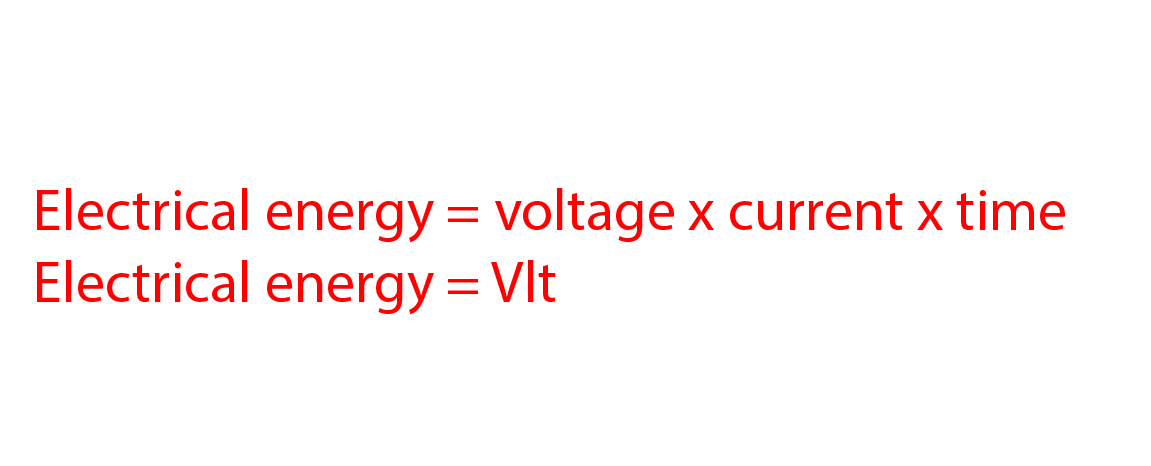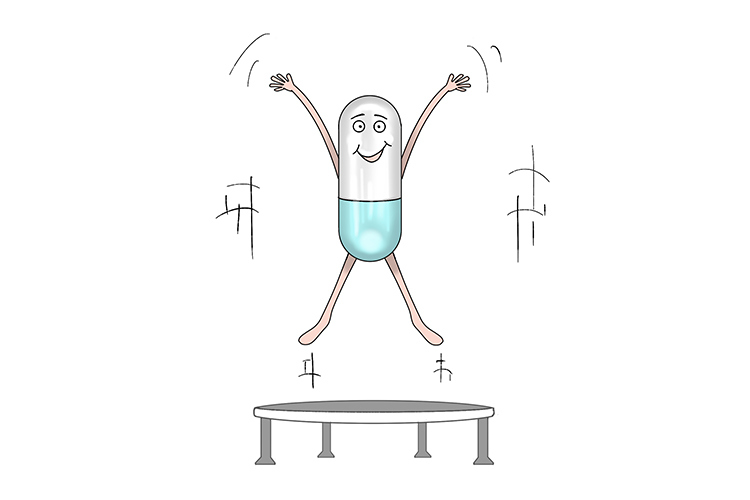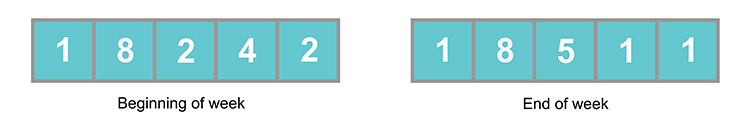# Electrical energy

Energy is what is needed for work to be done. In an electrical circuit, electrical energy is calculated using the formula:

Electrical energy = voltage x current x timeNo energy? You need vitamins! (VIt)

NOTE:

Energy is never created or destroyed but simply transformed or transferred from one type to another. In any system, when energy is transferred from one type to another not all of the energy is transferred to the useful type you may desire. Some of it may be lost as heat or light energy (due to friction) or sound, for instance. This is why no mechanical system can ever be 100% efficient. Energy is measured in Joules.

The amount of energy many electrical appliances use is often listed in kilowatt-hours (kWh). This is the unit in which domestic electricity is priced and is the amount of energy a 1kW appliance will use in one hour.

Energy used = power of appliance x time in use

(kWh)                         (kW)                   (in hours)

Example 1

Which of the following appliances will use the most energy?

A 100W lightbulb left on for 25 seconds

A 2.5kW electric heater in 30 minutes

A 8.5kW electric shower in 7.5 minutes

Energy = Power x time                  E = Pt

We must first convert everything to standard units, so:

2.5kW = 2500 W                30 minutes = 30 x 60 = 1800 seconds

8.5kW = 8500 W                7.5 minutes = 7.5 x 60 = 450 seconds

Energy used by lightbulb = 100 x 25 = 2500 Joules

Energy used by heater = 2500 x 1800 = 4,500,000 Joules

Energy used by shower = 8500 x 450 = 3,825,000 Joules

Therefore the heater is the appliance that uses the most energy.

Example 2

What is the power rating of an electric kettle that consumes 150kJ of energy per minute?

150kJ per minute = 150/60 = 2.5kJ per second = 2.5kW

Example 3

A domestic electric meter reading is shown in kWh below at the beginning and end of a week. If the electric company charges 13p for each kWh of electricity used:

1.  What is the total cost of the electricity used that week?

2.  How much energy would be used by a 2kW iron that’s used for 2.5hours?

3.  If a fifth of the electricity for that week was used by an electric car which has an average efficiency of 4 miles per kWh, how far could the car have travelled and what would this cost in electricity?1. 18511 – 18242 = 269kWh used that week

269 x 13 = 3497p = £34.97

2. Energy = Power x time                  E = Pt

Energy used by iron = 2 x 2.5 = 5kWh

3. 269kWh/5 = 53.8kWh

53.8 x 4 = 215.2miles

This would cost 53.8 x 13 = 699.4p = £6.99

Or £34.97/5 = £6.99